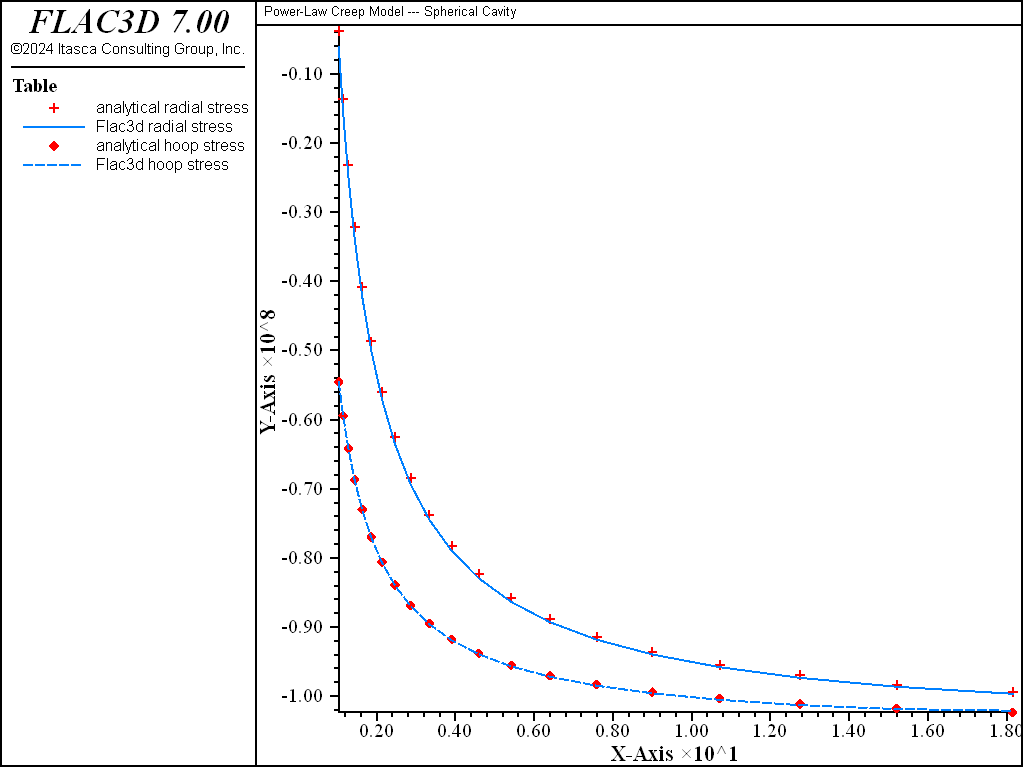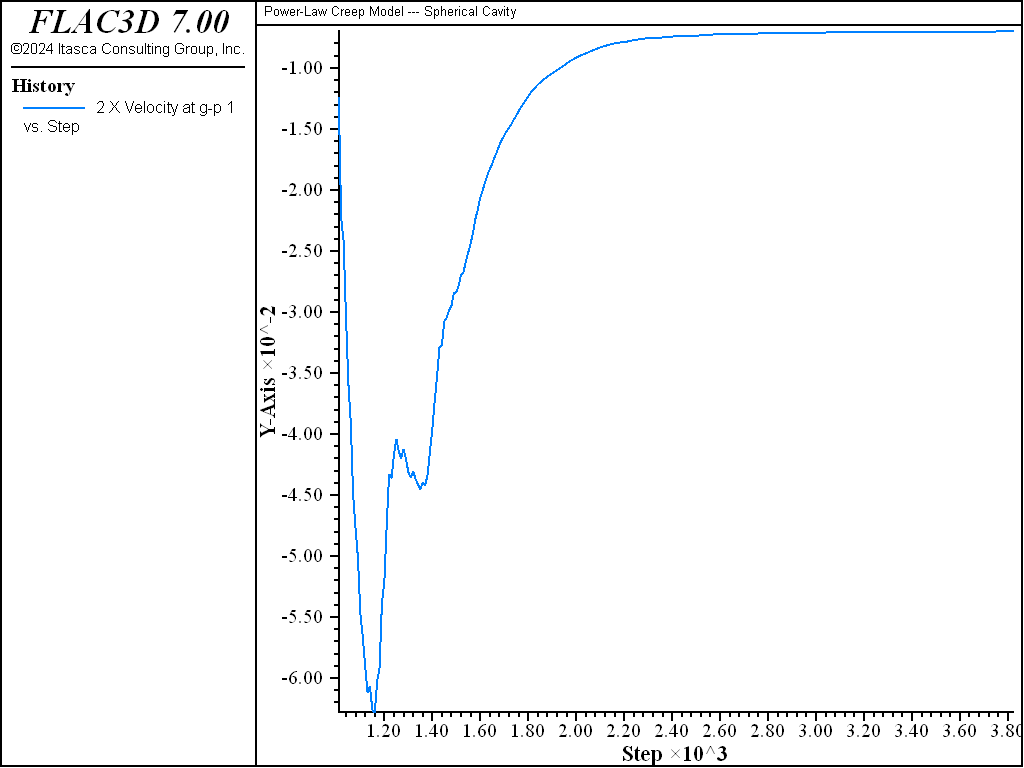# Power Model: Spherical Cavity

Note

To view this project in FLAC3D, use the menu command Help ► Examples…. Choose “Creep/SphericalCavityPower” and select “SphericalCavityPower.prj” to load. The project’s main data files are shown at the end of this example.

This problem is similar to Power Model: Cylindrical Cavity, but involves a thick sphere instead of a cylinder. The sphere is subject to a uniform pressure on its outer surface. The creep behavior is characterized by a single-component power law,

(1)$\dot \epsilon_{cr} = A \bar \sigma^n$

The analytical solution is given by van Sambeek (1986). The radial and hoop pressures are given by

$\begin{split}\begin{split} \sigma_{rr}&=-P_0 + {(P_0 - P_i) \over (b^{{-3 \over n}} - a^{{-3 \over n}})} \left[ b^{{-3 \over n}} - r^{{-3 \over n}}\right] \\ \sigma_{\phi\phi}&=\sigma_{\theta\theta} = -P_0 + {(P_0 - P_i) \over (b^{{-3 \over n}} - a^{{-3 \over n}})} \left[\left( {3 - 2n \over 2n} \right) r^{{-3 \over n}} + b^{{-3 \over n}}\right] \end{split}\end{split}$

(2)$\dot u = -{A \over 2} \left[{(P_0 - P_i) \left({-3 \over 2n}\right) \over (b^{{-3 \over n}} - a^{{-3 \over n}})} r^{{-3 \over n}} \right]^n r$

where $$P_0$$ and $$P_i$$ are the pressures at the inner and outer sphere surfaces, and $$a$$ and $$b$$ are the inner and outer radii, respectively.

The FLAC3D grid for this problem is shown in Figure 1. A single row of zones extending radially is used. The inner radius is at $$a$$ = 1, and the outer radius is at $$b$$ = 20.

Gridpoints are fixed in both circumferential directions, allowing only radial displacements. The pressure of 100 MPa was applied at the outer surface.

The material properties are

 $$A$$ = 1 × 10-7 MPa-3 yr-1 $$n$$ = 3 $$E$$ = 820 MPa $$\nu$$ = 0.3636

As in the cylindrical cavity case, an initial stress state of 100 MPa was assumed. Elastic equilibrium was reached first, then the creep calculation was initiated. The timestep started from a small value (10-4) and was allowed to increase up to the value set by model creep timestep maximum.

The results are summarized in Figure 2 through Figure 5. Figure 2 and Figure 3 compare the radial velocity and radial and hoop stresses obtained with FLAC3D with the analytical values.Figure 3: Comparison of radial and hoop stress at steady state—FLAC3D radial stress (symbol), analytical radial stress (line), FLAC3D hoop stress (symbol), analytical hoop stress (line) versus radial distance.

The histories of radial velocity at the cavity and the mechanical timestep are shown in Figure 4 and Figure 5, respectively. A behavior similar to that shown by the cylindrical cavity model in Power Model: Cylindrical Cavity is observed.Figure 4: History of radial velocity at the spherical cavity.

Data File

;------------------------------------------------------------
; power-law.f3dat
;        spherical cavity -- power law model
;-------------------------------------------------------------------
model new
model large-strain off
fish automatic-create off
model title "Power-Law Creep Model --- Spherical Cavity"
model configure creep

zone create brick  point 0 1 0 0               point 1 20 0 0           ...
point 3 0.98769 0.15643 0   point 6 19.754 3.129  0  ...
point 2 0.98769 0 -0.15643  point 4 19.754 0 -3.129  ...
point 5 0.97552 0.15643 -0.15451  ...
point 7 19.511 3.129 -3.090 ...
size 20 1 1  ratio 1.2 1 1

zone cmodel assign power
zone face skin
; Properties and stresses in Pascal units (not MPa)
zone property bulk=1e9 shear=3e8
zone property constant-1=1e-25 exponent-1=3
;
zone face apply velocity-normal 0 range group 'South'
zone face apply velocity-normal 0 range group 'North'
zone face apply velocity-normal 0 range group 'Bottom'
zone face apply velocity-normal 0 range group 'Top'
zone face apply stress-normal -100e6 range group 'East'
zone initialize stress xx -100e6 yy -100e6 zz -100e6
;
model creep active off
model step 1000
model save 'sph_1'
;
model history mechanical unbalanced-maximum
zone history velocity-x gridpointid 1
zone history velocity-x gridpointid 81
zone history displacement-x gridpointid 1
zone history displacement-x gridpointid 81
zone history stress-xx zoneid 1
zone history stress-yy zoneid 1
zone history stress-zz zoneid 1
model history creep time-total
model history timestep
;
zone gridpoint initialize velocity (0,0,0)
model creep active on
model creep timestep starting 1.0e-4
model creep timestep minimum 1.0e-4
model creep timestep maximum 1.0
;
model solve time-total 2000
model save 'sph_2'
;
program call 'AnalyticalSolution'  ; compare analytic solution
table '1'  label 'Flac3d'
table '11' label 'analytical'
table '2'  label 'Flac3d radial stress'
table '12' label 'analytical radial stress'
table '3'  label 'Flac3d hoop stress'
table '13' label 'analytical hoop stress'
model save 'power_law'
program return